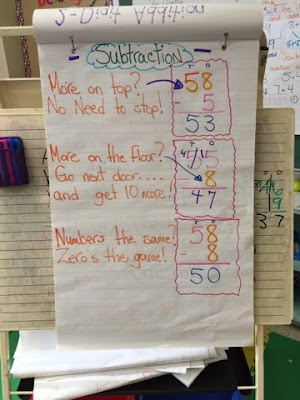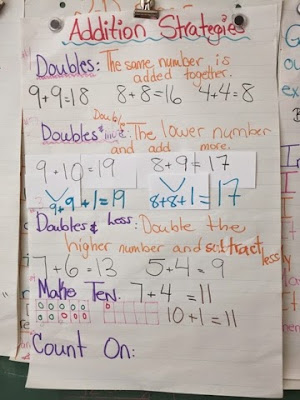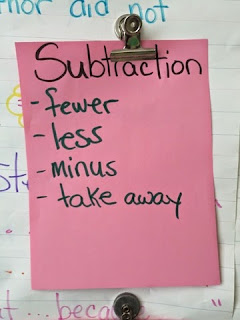Friday, April 03, 2015Over the past few weeks we have focused on Addition and Subtraction. We are working to add and subtract 3 digit numbers in grade 3. Students are learning many different strategies to help them to add and subtract without using their fingers. We are activating mental strategies by playing many different games.

We begin each Math lesson with a 2 minute math drill where we see how many facts we can solve in 2 minutes. We are also looking at some math images and finding addition and subtraction in pictures. Students are having fun looking at pictures in new ways to answer the simple question: What Math Do You See? Ask your child about it!

In addition, we came up with a few mental strategies to help us add numbers without using our fingers. Students are very comfortable with adding 2 digit numbers with and without carrying.With subtraction, students learned a catchy poem to help them recall how to subtract numbers. They understand subtracting much better when they are reminded of this poem. Please review this math poem with your children. This will really help them to remember how to subtract numbers! We will be moving to adding and subtracting 3 digit numbers next week, as well as focusing on money. The weekend homework practice will help them to review what they've learned this week. If you would like to challenge your children, you can give them 3 digit numbers to add or subtract and see if they remember what to do (for example: 123 + 421 OR 512 - 438).

We are also solving addition and subtraction problems using real life situations. Below are some key terms that you may see in a problem which will help students determine if they should use Addition or Subtraction. We will add more words to this list next week!